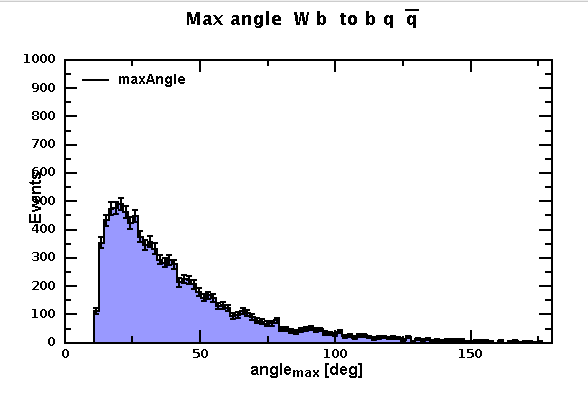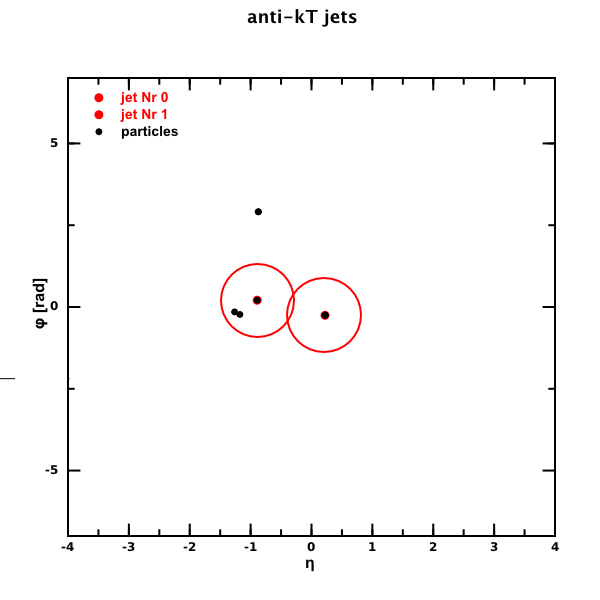# DMelt:Physics/HEP

Limitted access. First login to DataMelt if you are a full DataMelt member. Then login to HandWiki as a user.

# Showing data from HepData database

The best approach is to download "dmelt" Jython script from HepData database and run it in the DataMelt editor or as a batch script. This is the best supported approach which also allows to deal with statistical and systematical errors separately since data are imported to the P1D object. You can further modify P1D graphical attributes or replace HPlot canvas with other canvases (like HPlotJa). You can also combine statistical and systematic errors using the methods of the P1D class.

Here is a step-by step instruction:

• Go to Durham HepData database data. For example, click the link with the paper AAD 2013 — Search for new phenomena in photon+jet events collected in proton--proton collisions at sqrt(s) = 8 TeV.
• Navigate to "DataMelt" and use right-mouse and select "Copy link location"
• Start dmelt if you did not dod yet, and select [File]-[Read script from URL]. Copy and paster the URL link from the HepData database
• Click "run".

You will see an image with the HepData points.

You can read ROOT files with histograms or TGraph as discussed before. DataMelt use FreeHep Java API to read such files and convert to DataMelt native representation.

# Reading events with Monte Carlo simulation

DataMelt can be used to read and process Monte Carlo simulation events with particle collisions. The files can be download Monte Carlo events can be downloaded from HepSim Monte Carlo repository supported at the ANL laboratory. The HepSim also includes scripts illustrating how to access 4-momentum information on particles.

You can create a list with particles from HepSim and ProMC files usinghephysics.hepsim.PromcUtil class. You can create a lists for fast manipulation withhephysics.jet.FastParticle class, or list with the standardhephysics.particle.LParticle class.

Use DataMelt to read PDG table. Here is a handy script that reads the file "mass_width_2008.csv" located inside the DataMelt directory "macros/data".

This examples create "particle" objects with all needed informations (masses, spins, charges etc). The script assumes that you run it inside the DataMelt which defines the path to the mass_width_2008.csv file.

# Lorentz representation of a particle

You can represent a relativistic particle using a Lorentz representation. Use the classhephysics.particle.LParticle which defines a particle in terms of the four-Lorentz vector. A particle is characterized by either (px,py,pz,E) or (x,y,z,time).

For example, below we define a "top" quark with the mass 170 GeV and with the momentum pZ=400 GeV

from hephysics.particle import *
Top=LParticle("top quark",170)
Top.setV3( 0,0,400 )
print Top.toString()

Now we are ready to go with a small Monte Carlo simulation for decay of the top particle. Below we calculate the maximum opening angle between its decay products

from java.awt import Color
from jhplot  import *
from java.util import Random
from hephysics.particle import *
from math import *

P=400
# define initial  Lorentz particle
Top=LParticle("Top quark",170)
Top.setV3( 0,0,P )
print Top.toString()

# define Lorentz particles
W = LParticle("W",80)
b = LParticle("b",5)
q1 = LParticle("q1",0.004)
q2 = LParticle("q2",0.004)

r = Random()
PI=3.1415926
PI2=2*PI
deg= 180 / PI

h1 = H1D("maxAngle",100, 0,180)
for i in range(10000):
if (i%1000==0): print "event=",i

Top.twoBodyDecay(W, b,1);
theta = acos(2.0*r.nextDouble()-1.0 )
phi   = PI2 *r.nextDouble()
Top.setThetaPhiP(theta,phi,P)
W.boost( Top )
b.boost( Top )
# W decay
W.twoBodyDecay(q1,q2,1)
# the boost
q1.boost( W )
q2.boost( W )

# print q1.toString()
p=P0D("max angle")
pmax=p.getMax()
h1.fill(pmax)

c1 = HPlot("Canvas",600,400,1, 1)
c1.setGTitle("Max angle t to W b to b q #bar{q}")
c1.visible(1)
c1.setRange(0,180,0,1000)
c1.setNameX("angle_{max} [deg]")
c1.setNameY("Events")

h1.setFill(1)
h1.setFillColor(Color.blue)
c1.draw(h1)

# export to EPS figure
# c1.export("angle"+".eps")

The output of the code is below:# Feynman diagrams

One can draw Feynman diagrams programically using Java, Python (Jython) or any scripting language supported by DataMelt. Look at the section Drawing diagrams which shows how to draw diagrams and export to a number of image formats.

DataMelt includes Redberry computer algebra (CA) system designed for algebraic manipulations with tensors. It supports symbolic algebra, tensor-specific transformations, simplification, Feynman diagrams and one-loop counterterm. Read "Introduction to Redberry: the computer algebra system designed for tensor manipulation" by D. A. Bolotin and S. V. Poslavsky (arXiv:1302.1219 [cs.SC]).

Currently, the description of Redberry is beyond the scope of this manual. You can run the Groovy tutorials that are given in the documentation.

# Jet algorithms

DataMelt includes a number of jet algorithms implemented in Java. This is a list of the algorithms:

It is recommended to usehephysics.jet.JetN2 jet algorithm since it shows the best performance, similar to the FastJet C++ program. The algorithm was implemented by I.Pogrebnyak. See hepjet package for benchmark results.

Thehephysics.jet.KTjet class is interfaces with the LParticle class. You can run the longitudinally invariant kT algorithm in inclusive mode. Jet can be clustered using Cambridge/Aachen or anti-kT approaches. It uses Eta-Phi space to make jets (not Rapidity-Phi). Look at theKT jet package summary.

Let us give an example of how to visualize anti-kT jets in Eta-Phi plane using Jython script:

from java.awt import Color
from java.util import ArrayList
from jhplot  import *
from hephysics.jet import *
from hephysics.particle import *
from jhplot.shapes import *

plist=ArrayList()

R=0.6 # jet radius. Use anti-kT jets
ktjet = SCJet(R, 1, -1, 200.0,True);
ktjet.buildJets(plist);
ktjet.printJets()
jets=ktjet.getJetsSorted()

c1 = HPlot("Canvas",600,600)
c1.setGTitle("anti-kT jets")
c1.visible(1)
c1.setRange(-4,4,-7,7)
c1.setNameX("&eta;")

pp=P1D("particles")

for i in range(plist.size()):
p=plist.get(i)
eta=p.getRapidity()
phi=p.getPhi()
# psize=int(p.getEt()/10.)
# pp.setSymbolSize(2)

# show jets
for k in range(jets.size()):
jet=P1D("jet Nr "+str(k))
jet.setColor(Color.red)
jet.setSymbolSize(7)
jj=jets.get(k)
etaJ=jj.getRapidity()
phiJ=jj.phi()
c1.draw(pp)